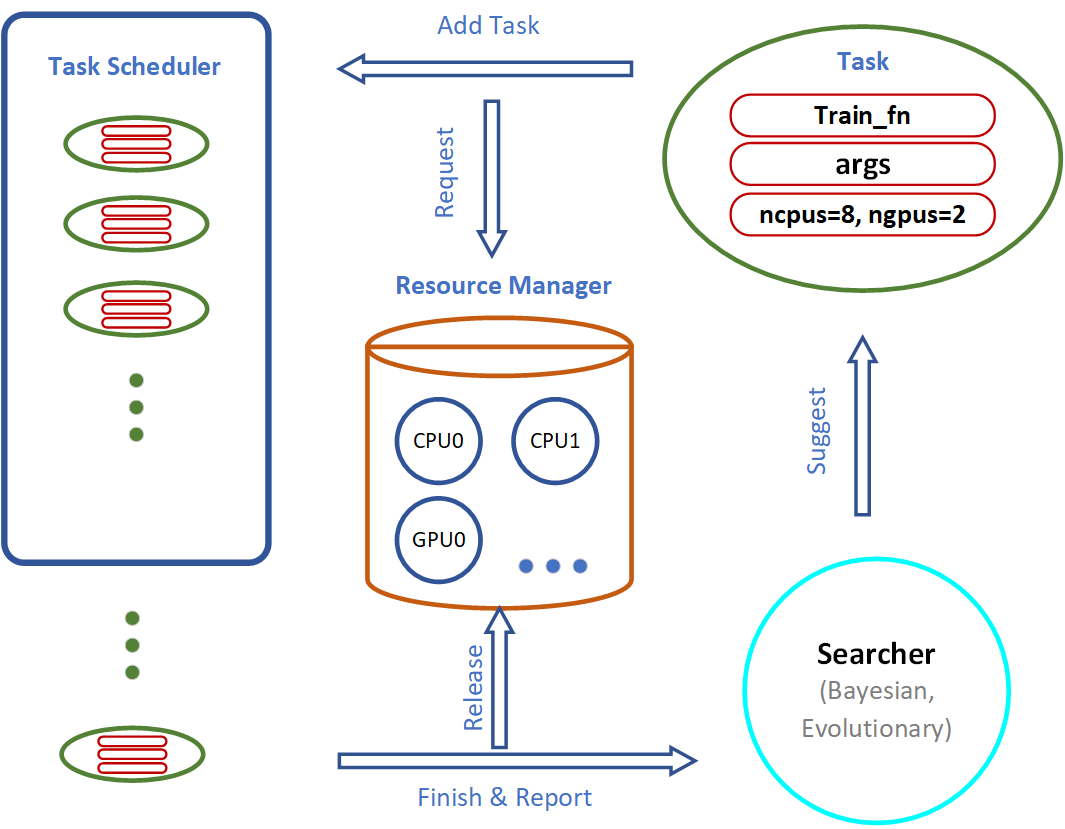# Search Algorithms¶

## AutoGluon System Implementation Logic¶Important components of the AutoGluon system include the Searcher, Scheduler and Resource Manager:

• The Searcher suggests hyperparameter configurations for the next training job.

• The Scheduler runs the training job when computation resources become available.

In this tutorial, we illustrate how various search algorithms work and compare their performance via toy experiments.

## FIFO Scheduling vs. Early Stopping¶

In this section, we compare the different behaviors of a sequential First In, First Out (FIFO) scheduler using autogluon.core.scheduler.FIFOScheduler vs. a preemptive scheduling algorithm autogluon.core.scheduler.HyperbandScheduler that early-terminates certain training jobs that do not appear promising during their early stages.

### Create a Dummy Training Function¶

import numpy as np
import autogluon.core as ag

@ag.args(
lr=ag.space.Real(1e-3, 1e-2, log=True),
wd=ag.space.Real(1e-3, 1e-2),
epochs=10)
def train_fn(args, reporter):
for e in range(args.epochs):
dummy_accuracy = 1 - np.power(1.8, -np.random.uniform(e, 2*e))
reporter(epoch=e+1, accuracy=dummy_accuracy, lr=args.lr, wd=args.wd)


### FIFO Scheduler¶

This scheduler runs training trials in order. When there are more resources available than required for a single training job, multiple training jobs may be run in parallel.

scheduler = ag.scheduler.FIFOScheduler(train_fn,
resource={'num_cpus': 2, 'num_gpus': 0},
num_trials=20,
reward_attr='accuracy',
time_attr='epoch')
scheduler.run()
scheduler.join_jobs()

  0%|          | 0/20 [00:00<?, ?it/s]


Visualize the results:

scheduler.get_training_curves(plot=True, use_legend=False)


### Hyperband Scheduler¶

AutoGluon implements different variants of Hyperband scheduling, as selected by type. In the stopping variant (the default), the scheduler terminates training trials that don’t appear promising during the early stages to free up compute resources for more promising hyperparameter configurations.

scheduler = ag.scheduler.HyperbandScheduler(train_fn,
resource={'num_cpus': 2, 'num_gpus': 0},
num_trials=100,
reward_attr='accuracy',
time_attr='epoch',
grace_period=1,
reduction_factor=3,
type='stopping')
scheduler.run()
scheduler.join_jobs()

  0%|          | 0/100 [00:00<?, ?it/s]


In this example, trials are stopped early after 1, 3, or 9 epochs. Only a small fraction of the most promising jobs run for the full number of 10 epochs. Since the majority of trials are stopped early, we can afford a larger num_trials. Visualize the results:

scheduler.get_training_curves(plot=True, use_legend=False)


Note that HyperbandScheduler needs to know the maximum number of epochs. This can be passed as max_t argument. If it is missing (as above), it is inferred from train_fn.args.epochs (which is set by epochs=10 in the example above) or from train_fn.args.max_t otherwise.

## Random Search vs. Reinforcement Learning¶

In this section, we demonstrate the behaviors of random search and reinforcement learning in a simple simulation environment.

### Create a Reward Function for Toy Experiments¶

Import the packages:

import matplotlib.pyplot as plt
from mpl_toolkits.mplot3d import Axes3D


Input Space x = [0: 99], y = [0: 99]. The rewards is a combination of 2 gaussians as shown in the following figure:

Generate the simulated reward as a mixture of 2 gaussians:

def gaussian2d(x, y, x0, y0, xalpha, yalpha, A):
return A * np.exp( -((x-x0)/xalpha)**2 -((y-y0)/yalpha)**2)

x, y = np.linspace(0, 99, 100), np.linspace(0, 99, 100)
X, Y = np.meshgrid(x, y)

Z = np.zeros(X.shape)
ps = [(20, 70, 35, 40, 1),
(80, 40, 20, 20, 0.7)]
for p in ps:
Z += gaussian2d(X, Y, *p)


Visualize the reward space:

fig = plt.figure()
ax = fig.gca(projection='3d')
ax.plot_surface(X, Y, Z, cmap='plasma')
ax.set_zlim(0,np.max(Z)+2)
plt.show()

/var/lib/jenkins/workspace/workspace/autogluon-tutorial-course-v3/venv/lib/python3.7/site-packages/ipykernel_launcher.py:2: MatplotlibDeprecationWarning: Calling gca() with keyword arguments was deprecated in Matplotlib 3.4. Starting two minor releases later, gca() will take no keyword arguments. The gca() function should only be used to get the current axes, or if no axes exist, create new axes with default keyword arguments. To create a new axes with non-default arguments, use plt.axes() or plt.subplot().


### Create Training Function¶

We can simply define an AutoGluon searchable function with a decorator ag.args. The reporter is used to communicate with AutoGluon search and scheduling algorithms.

@ag.args(
x=ag.space.Categorical(*list(range(100))),
y=ag.space.Categorical(*list(range(100))),
)
def rl_simulation(args, reporter):
x, y = args.x, args.y
reporter(accuracy=Z[y][x])


### Reinforcement Learning¶

rl_scheduler = ag.scheduler.RLScheduler(rl_simulation,
resource={'num_cpus': 1, 'num_gpus': 0},
num_trials=300,
reward_attr="accuracy",
controller_batch_size=4,
controller_lr=5e-3)
rl_scheduler.run()
rl_scheduler.join_jobs()
print('Best config: {}, best reward: {}'.format(rl_scheduler.get_best_config(), rl_scheduler.get_best_reward()))

100%|██████████| 76/76 [03:09<00:00,  2.49s/it]

Best config: {'x▁choice': 21, 'y▁choice': 74}, best reward: 0.9892484241569526


### Compare the performance¶

Get the result history:

results_rl = [v['accuracy'] for v in rl_scheduler.training_history.values()]
results_random = [v['accuracy'] for v in random_scheduler.training_history.values()]


Average result every 10 trials:

import statistics
results1 = [statistics.mean(results_random[i:i+10]) for i in range(0, len(results_random), 10)]
results2 = [statistics.mean(results_rl[i:i+10]) for i in range(0, len(results_rl), 10)]


Plot the results:

plt.plot(range(len(results1)), results1, range(len(results2)), results2)

[<mpl_toolkits.mplot3d.art3d.Line3D at 0x7f5d2e17bc90>,
<mpl_toolkits.mplot3d.art3d.Line3D at 0x7f5cbe8bd250>]